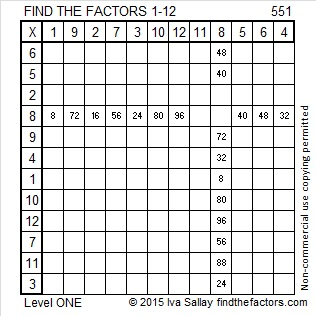# 551 and Level 1

551 is the sum of all the prime numbers from 11 to 67. It also equals this sum of consecutive primes: 179 + 181 + 191.

551 is the hypotenuse of the Pythagorean triple 380-399-551. What is the greatest common factor of those three numbers? Hint: It’s one of the factors of 551 listed below the puzzle.Print the puzzles or type the solution on this excel file: 12 Factors 2015-07-13

—————————————————————————————————

• 551 is a composite number.
• Prime factorization: 551 = 19 x 29
• The exponents in the prime factorization are 1 and 1. Adding one to each and multiplying we get (1 + 1)(1 + 1) = 2 x 2 = 4. Therefore 551 has exactly 4 factors.
• Factors of 551: 1, 19, 29, 551
• Factor pairs: 551 = 1 x 551 or 19 x 29
• 551 has no square factors that allow its square root to be simplified. √551 ≈ 23.453389—————————————————————————————————This site uses Akismet to reduce spam. Learn how your comment data is processed.